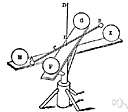# gravitational constant

(redirected from Gravity constant)
Also found in: Thesaurus, Medical, Encyclopedia.
Related to Gravity constant: Acceleration due to gravity

## gravitational constant

n. Abbr. G
The constant relating the force of gravitational attraction between two bodies to the product of their masses and the inverse square of the distance between them in Newton's law of gravitation. It equals 6.67 × 10-11 m3kg-1s-2.

## gravitational constant

n
(General Physics) the factor relating force to mass and distance in Newton's law of gravitation. It is a universal constant with the value 6.673 × 10–11 N m2 kg–2. Symbol: G

## grav·i·ta·tion·al constant

(grăv′ĭ-tā′shə-nəl)
A number used to calculate the force of the gravitational attraction between two bodies in Newton's law of gravitation. The gravitational constant equals 6.67 × 10-11 cubic meters per kilogram per second squared. See more at Newton's law of gravitation.
ThesaurusAntonymsRelated WordsSynonymsLegend:
 Noun 1gravitational constant - (physics) the universal constant relating force to mass and distance in Newton's law of gravitationlaw of gravitation, Newton's law of gravitation - (physics) the law that states any two bodies attract each other with a force that is directly proportional to the product of their masses and inversely proportional to the square of the distance between themnatural philosophy, physics - the science of matter and energy and their interactions; "his favorite subject was physics"constant - a number representing a quantity assumed to have a fixed value in a specified mathematical context; "the velocity of light is a constant"
Translations
Gravitationskonstante
Site: Follow: Share:
Open / Close